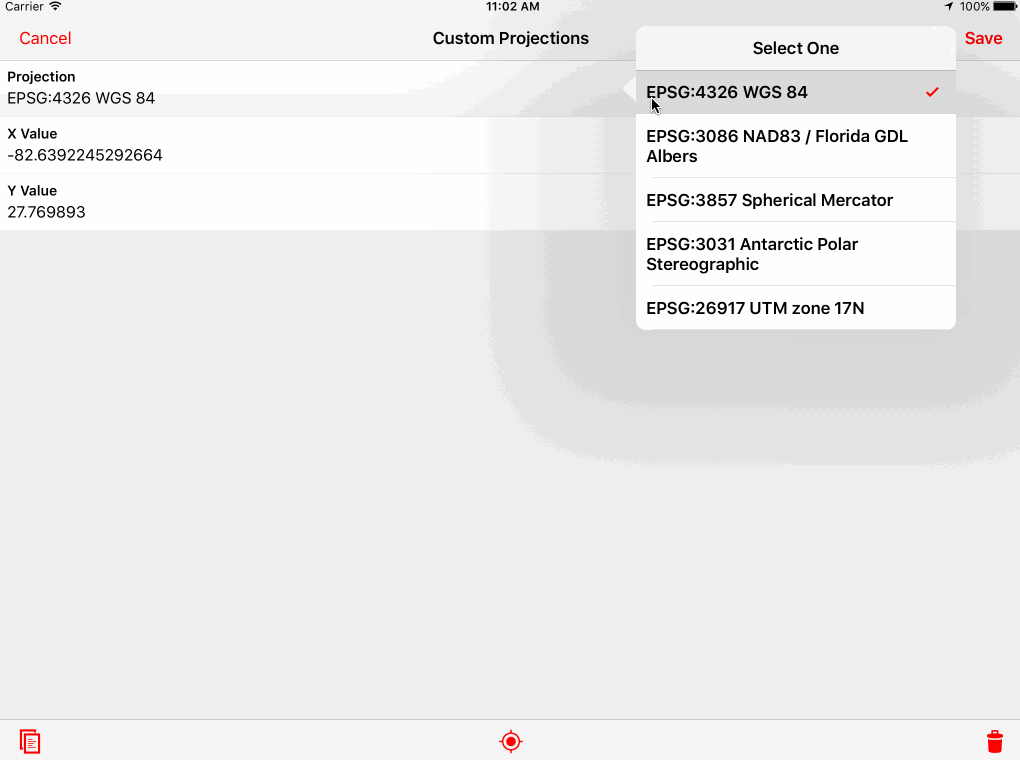Using Custom Projections in Calculation Fields

# Using Custom Projections in Calculation Fields

Earlier this year we launched calculation fields, and we’re constantly impressed with the ways our customers are using them to add value to their field surveys. I want to demo a neat technique you can use to embed proj4js into a calculation field to gain access to custom GIS projections.

First, I’ll just show you the result:The idea in the demo is pretty simple. The app uses the `LATITUDE()` and `LONGITUDE()` functions to display and store the record location using different projections in separate calculation fields. In the demo app, the projection can also be changed on the fly using a choice field. Here is a table of the projections used in the demo app:

## Projections

EPSG Code Proj.4 Text
EPSG:4326 WGS 84 `+proj=longlat +datum=WGS84 +no_defs`
EPSG:3086 NAD83 / Florida GDL Albers `+proj=aea +lat_1=24 +lat_2=31.5 +lat_0=24 +lon_0=-84 +x_0=400000 +y_0=0 +ellps=GRS80 +datum=NAD83 +units=m +no_defs`
EPSG:3857 Spherical Mercator `+proj=merc +a=6378137 +b=6378137 +lat_ts=0.0 +lon_0=0.0 +x_0=0.0 +y_0=0 +k=1.0 +units=m +nadgrids=@null +wktext +no_defs`
EPSG:3031 Antarctic Polar Stereographic (just for fun) `+proj=stere +lat_0=-90 +lat_ts=-71 +lon_0=0 +k=1 +x_0=0 +y_0=0 +datum=WGS84 +units=m +no_defs`
EPSG:26917 UTM zone 17N `+proj=utm +zone=17 +ellps=GRS80 +towgs84=0,0,0,0,0,0,0 +units=m +no_defs`

## Including the JavaScript library

To access the external proj4js library in multiple calculation fields, I’ve added a calculation field to the form that just includes the proj4js library. Bear with me here because this is a bit of a “workaround” to attach some code to use in multiple calculation fields. One important thing to remember with calculation fields is that everything in calculation field expressions is “just Javascript”. On Android, expressions are evaluated using an embedded version of the V8 JavaScript Engine and on iOS Fulcrum uses JavaScriptCore. These are the same JavaScript engines Chrome and Safari use. So most pure JavaScript libraries will work, but might require some tweaks depending on how the library is implemented.

Here is the source of the “Proj4js” calculation field that exports a `proj4` function we can use in other calculation fields.

Once we have this calculation field setup, other calculation fields can use the library with `this.proj4`. The technique of attaching code to `this` in order to share it across calculation fields is important. Otherwise we would have to duplicate the entire proj4js library in each calculation field we wanted to use the library in.

Here is the code to use the main proj4js function to calculate the X value using a different projection:

It starts by pulling out the projection from the `projection` choice field on the form. For demonstration purposes, the app lets you select the projection you want. The `proj4Text` variable stores the proj4 text. You could also hardcode this text in the calculation for whatever projection you care about. If you aren’t familiar with proj4, that’s OK, you can use epsg.io or spatialreference.org to find the proj4 text for almost any projection.

To calculate the Y value, it uses a nearly identical expression except it sets the result to `result` instead of `result`. You can find more documentation on how to use proj4js on their website. If you just want to silently capture the projected X/Y values, you can hide all of the calculation fields and all of this will be invisible during the surveying.

## Try it Yourself

Here is a link to the demo app in the app gallery. You can add it to your account to try it out yourself.

You can also use this same technique to add other Javascript libraries to Fulcrum calculation fields to do interesting things with your data. Check back for more posts on how to do neat tricks with calculation fields.